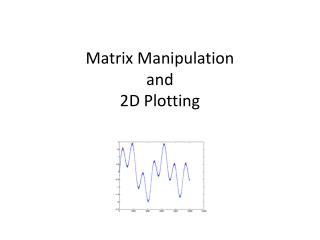# Matrix Manipulation and 2D Plotting - PowerPoint PPT PresentationDownload PresentationMatrix Manipulation and 2D Plotting

Matrix Manipulation and 2D Plotting
Download Presentation## Matrix Manipulation and 2D Plotting

- - - - - - - - - - - - - - - - - - - - - - - - - - - E N D - - - - - - - - - - - - - - - - - - - - - - - - - - -
##### Presentation Transcript

1. Matrix Manipulationand2D Plotting

2. Review • Command Line • Semi Colon to suppress output • X = 3; • Entering a Matrix • Square brackets • X = [1,2,3,4,5] • Generate arrays (1D matrices) • X = Start:Step:End • X = 1:3:13

3. Matrix Manipulation • The basic unit of data storage • Extract a part of a matrix use round brackets • third entry in a 1D matrix • X(3) • last Entry • X(end) • fourth to ninth entries • X(4:9) • A 2D matrix • Entry at the third row, second column • A(3,2) • All of the third row • A(3,:)

4. Examples • Create a 5 by 5 magic square • There is a inbuilt function • Extract the value at the third row, fourth column • Extract the last value of the third column • Extract all of the fifth row • Replace the value at row 2, column 4 with 100 • Replace all of the first column with zeros • Delete all of the fourth row A = magic(5) A(3,4) A(end,3) A(5,:) A(2,4)=100 A(:,1)=0 A(4,:)=[]

5. Questions • What do the following command do? • If A is a 2D matrix • A' • B = [A';A'] • A(:)

6. Solving linear Systems • Represent in matrix form • Use backslash operator • Inv(A)*b • A/b

7. Solve

8. 2D Plotting • Various, flexible, plotting routines. • The basic command is • plot(x,y) • This plots the vector of x coordinates against the vector of y coordinates • X and y must be the same size • plot(y) • Plots the vector y against its indices

9. Plotting • Plot the function y = sin(x) +x -2 • for x = [-2,9] x= -2:0.1:9; y = sin(x)+ x-2; plot(x,y)

10. Plot the following • y = 0.2* e-0.1x • for x = [-1,1] • y = sin(x) +x^2 -2 • for x = [-5,10]

11. Data Loading • Navigate to appropriate directory • Right click on file and select importdata • If in plain text format • A = Load(‘file name.???’); • If a other formats (eg. Excel files) • A = importdata(‘file name.xls’);

13. >> data = load('c:/CVBulk.tsv'); >> figure >> plot(data(:,1),data(:,2),'r') >> title('CV') >> xlabel('Voltage / V') >> ylabel('Current / A')

14. Changing Attributes • Basic usage: plot(x, y, ’Attributes’) • To plot a green dashed line • plot(x, y, ’g--’) • To plot yellow circle at the data points • plot(x, y, ‘yo’)

15. Titles etc • Adding a title • Title(‘whoop whoop’) • Axes labels • xlabel(‘Peanuts’) • ylabel(‘Vanilla’) • Legend • legend('222','33')

16. Multiple • To plot multiple line of the same plot • plot(X,Y,'y-',X1,Y1,'go') • Or use the ‘hold on’ function • plot(x, y, ’b.’) • hold on • plot(v, u, ‘r’)

17. Subplot • Used to plot multiple graphs in the same frame • Try: • Plot sine and cosine between 0 and 10 on two separate axes in the same frame >> subplot(2,1,1) >> plot(x, y) >> subplot(2,1,2) >> plot(u, v)

18. More attributes: • Plots are fully editable from the figure window. Once you have a plot, you can click Tools->Edit plot to edit anything. • plot(X1,Y1,LineSpec,'PropertyName',PropertyValue) • Plot(x, y, ’r’, ‘LineWidth’, 3, ‘MarkerSize',10) • But all this can also be done from the command line • set(gca,'XTick',-pi:pi/2:pi)

19. More types of plots • Histograms • Bar • Pie Charts • Create a bar plot of the CVBulk data

20. Task • Plot the following equations on the same graph (different colours) • Solve the system of equations • Plot the solution on the same graph • Add appropriate titles, labels and legend